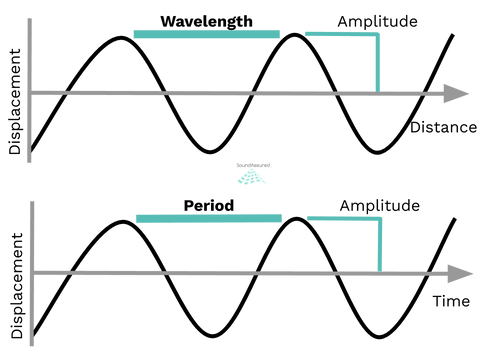# What Are Sound Waveform Characteristics ?A waveform is the graphical representation of a signal or sound as it moves through a medium over time. A waveform generally has the following characteristics allowing them to be distinguished from each other.
• Amplitude
• Frequency
• Wavelength & Period

Amplitude is the distance above or below the centerline or the x-axis. The greater the distance away from the centerline the more intense the signal.Frequency is the rate at which the signal repeats a 360 degree cycle of positive and negative amplitude over a period of time. Sound waves are calculated using a period of one second and are measure in hertz. More cycles or repeated vibrations within a given period of time indicate a higher frequency.Wavelength is the measure of displacement over distance.

Period is the measure of displacement over time

Wavelength is the physical distance between the start and finish of one wave cycle and can be calculated using mathematical formulas.Other characteristics of sound include:

• Velocity
• Phase
• Harmonics
• Octaves

Velocity is the measure of how fast the sound wave travels through the air . It is basically the speed of sound and changes as temperature goes up and down.

The velocity (speed) of a sound in normal temperature is about 770 miles per hour. This speed is commonly know as "Mach 1.0" in the field of aerodynamics. For reference, a Boeing 787 travels at Mach 0.85.

It takes sound approximately 5 seconds to travel one mile. This may seem fast, but compared to light the speed of sound is slow! Light can travel one mile in just 5.3 microseconds!

Phase is an exact position at a point of time of a waveform. Phase is used to describe a single location within a particular wavelength. If two waveforms have the same frequency, shape, and amplitude they are considered to be in phase.

Harmonics

Harmonics represent integral multiples of a fundamental frequency.

For example, if the fundamental frequency is 220 Hz, A3 note on the Piano, the frequencies of the first three higher harmonics are 440 Hz (2nd harmonic), 660 Hz (3rd harmonic), 880 Hz (4th harmonic).

Octaves

On octave is the interval between two frequencies having a ratio of 2:1.

For example, look at the A3 note. As is 220 Hz. A4 is considered one octave up and has a frequency of 440 Hz. A2 is considered one octave down from A3 and has a frequency of 110 Hz.

--
--

What Is Transmission Loss Of Sound ?

What Is Noise Reduction Coefficient (NRC) ?

Acoustic Foam Room Calculator

Will Acoustic Foam Soundproof A Room ?

Acoustic Foam FAQ

How To Cut Acoustic Foam

Acoustic Treatment For Home Recording Studios - Multiple Design Ideas